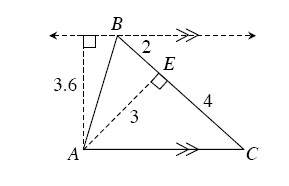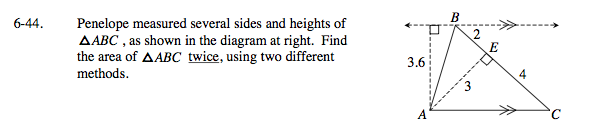Home > GC > Chapter 6 > Lesson 6.2.1 > Problem6-44

6-44.
1. Penelope measured several sides and heights of ΔABC , as shown in the diagram below. Find the area of ΔABC twice, using two different methods. Homework Help ✎

2.$A=\frac{1}{2}bh$

Remember: a base and a height must be perpendicular!
Which side can be used as a base? As the height?

$\text{One method could be to use }\overline{BC}\text{ as the}$

$\text{base and }\overline{AE}\text{ as the height.}$

$\text{Area = }{\frac{1}{2}}(6)(3)=9$

$\text{Use another side as a base. But why is using }\overline{AB}\text{ as the base a bad idea?}$

$\text{Use }\overline{AC}\text{ as the base.}$